• 改写二分搜索算法
2021-10-09 16:44:43

题目来源：《计算机算法设计与分析》，王晓东

设a[0:n-1]是已排好序的数组，请改写二分搜索算法，使得当x不在数组中时，返回小于x的最大元素位置i和大于x的最小元素位置j。当搜索元素在数组中时，i和j相同，均为x在数组中的位置。

### 输入格式:

输入有两行：

第一行是n值和x值； 第二行是n个不相同的整数组成的非降序序列，每个整数之间以空格分隔。

### 输出格式:

输出小于x的最大元素的最大下标i和大于x的最小元素的最小下标j。当搜索元素在数组中时，i和j相同。 提示：若x小于全部数值，则输出：-1 0 若x大于全部数值，则输出：n-1的值 n的值

输入样例:
在这里给出一组输入。
例如：
6 5
2 4 6 8 10 12
输出样例:
在这里给出相应的输出。
例如：1 2

#include<iostream>
using namespace std;
void changeBinarySearch(int a[],int l,int r,int x)
{
if(l==r){
if(a[l]<x){
cout<<l<<" "<<l+1;
}
if(a[l]==x)cout<<l<<" "<<l;
if(a[l]>x)cout<<l-1<<" "<<l;
return;
}
if(l>r)
{
cout<<l<<" "<<l+1;
}
int m=(r+l)/2;
if(a[m]==x){
cout<<m<<" "<<m;
}
else if(a[m]>x){
return changeBinarySearch(a,l,m-1,x);
}
else
{
return changeBinarySearch(a,m+1,r,x);
}
}
int main()
{
int n,a,x;
cin>>n;
cin>>x;
for(int i=0;i<n;i++)
{
cin>>a[i];
}
if(x<a){
cout<<-1<<" "<<0;
}
else if(x>a[n-1]){
cout<<n-1<<" "<<n;
}
else changeBinarySearch(a,0,n-1,x);
system("pause");
}数据结构
更多相关内容
• 设a[0:n-1]是一个已排好序的数组。请改写二分搜索算法，使得当搜索元素x不在数组中时，返回小于x的最大元素的位置I和大于x的最大元素位置j。
• 设a[0:n-1]是已排好序的数组，请改写二分搜索算法，使得当搜索元素x不在数组中时，返回小于x的最大元素位置i和大于x的最大元素位置j。当搜索元素在数组中时，i和j相同，均为x在数组中的位置。 下面是将要被改写的二...

# 设a[0:n-1]是已排好序的数组，请改写二分搜索算法，使得当搜索元素x不在数组中时，返回小于x的最大元素位置i和大于x的最大元素位置j。当搜索元素在数组中时，i和j相同，均为x在数组中的位置。

下面是将要被改写的二分搜索算法

   public static int binarySearch1(int []a,int x,int n){
int left = 0;int right = n-1;
while(left<=right){
int middle = (left+right)/2;
if(x==a[middle]) return middle;
if(x>a[middle]) left= middle-1;
else right = middle;
}
return -1;
}


改写后的二分搜索算法

public class BinarySearch {
public static int[] binarySearch(int[] a, int x, int left,int right, int[] b){
int i;int j;
while(left<=right){
int middle = ( left + right ) / 2;
if (x==a[middle]){
i=j=middle;
b=i;
b=j;
return b;
}
if (x>a[middle])
left=middle+1;
else
right=middle-1;
}
i=right;j=left;
b=i;
b=j;
return b;
}
public static void main(String[] args) {
int []a= {1, 2, 3, 4, 5,6, 7,8, 9};
int n=a.length;int i=0; int j=0;
int left=0;int right=n-1;
int []b=new int;
int x=-1;
binarySearch(a,x,left,right,b);
if (x<a[left]){
System.out.println("x比数组的最小值还要小，大于x的最小元素的位置位于数组中的第1位");
}else if(x<=a[right]){
if (b==b)
System.out.println("该元素位于数组中的第"+(b+1)+"个");
else {
System.out.println("该元素不在数组中");
System.out.println("小于x的最大元是第"+(b+1)+"个");
System.out.println("大于x的最小元是第"+(b+1)+"个");
}
}else{
System.out.println("x比数组的最大值还要大，小于x的最大元素的位置位于数组中的第"+(n+1)+"位");
}
}
}


展开全文java
• 设a[0:n-1]是已排好序的数组，请改写二分搜索算法，使得当x不在数组中时，返回小于x的最大元素位置i和大于x的最小元素位置j。当搜索元素在数组中时，i和j相同，均为x在数组中的位置。 输入格式: 输入有两行： 第一行...

题目来源：《计算机算法设计与分析》，王晓东

设a[0:n-1]是已排好序的数组，请改写二分搜索算法，使得当x不在数组中时，返回小于x的最大元素位置i和大于x的最小元素位置j。当搜索元素在数组中时，i和j相同，均为x在数组中的位置。

输入格式:
输入有两行：

第一行是n值和x值； 第二行是n个不相同的整数组成的非降序序列，每个整数之间以空格分隔。

输出格式:
输出小于x的最大元素的最大下标i和大于x的最小元素的最小下标j。当搜索元素在数组中时，i和j相同。 提示：若x小于全部数值，则输出：-1 0 若x大于全部数值，则输出：n-1的值 n的值

输入样例:
在这里给出一组输入。例如：

6 5
2 4 6 8 10 12
输出样例:
在这里给出相应的输出。例如：

1 2

#include<stdio.h>
int search(int x,int a[],int left,int right){
int center=(left+right)/2;
if(a[center-1]<x && x<a[center])
printf("%d %d\n",center-1,center);
else if(left<=right){
if(x==a[center])
printf("%d %d\n",center,center);
else if(x<a[center])
search(x,a,left,center-1);
else if(x>a[center])
search(x,a,center+1,right);
}
return 0;
}
int main(){
int n,x;
int a;
scanf("%d %d",&n,&x);
for(int i=0;i<n;i++){
scanf("%d",&a[i]);
}
if(x<a)
printf("-1 0\n");
else if(x>a[n-1])
printf("%d %d\n",n-1,n);
else search(x,a,0,n-1);
return 0;
}

展开全文c语言
• 改写二分搜索算法。返回小于x的最大元素位置和大于x的最小元素位置。

### 改写二分搜索算法

题目：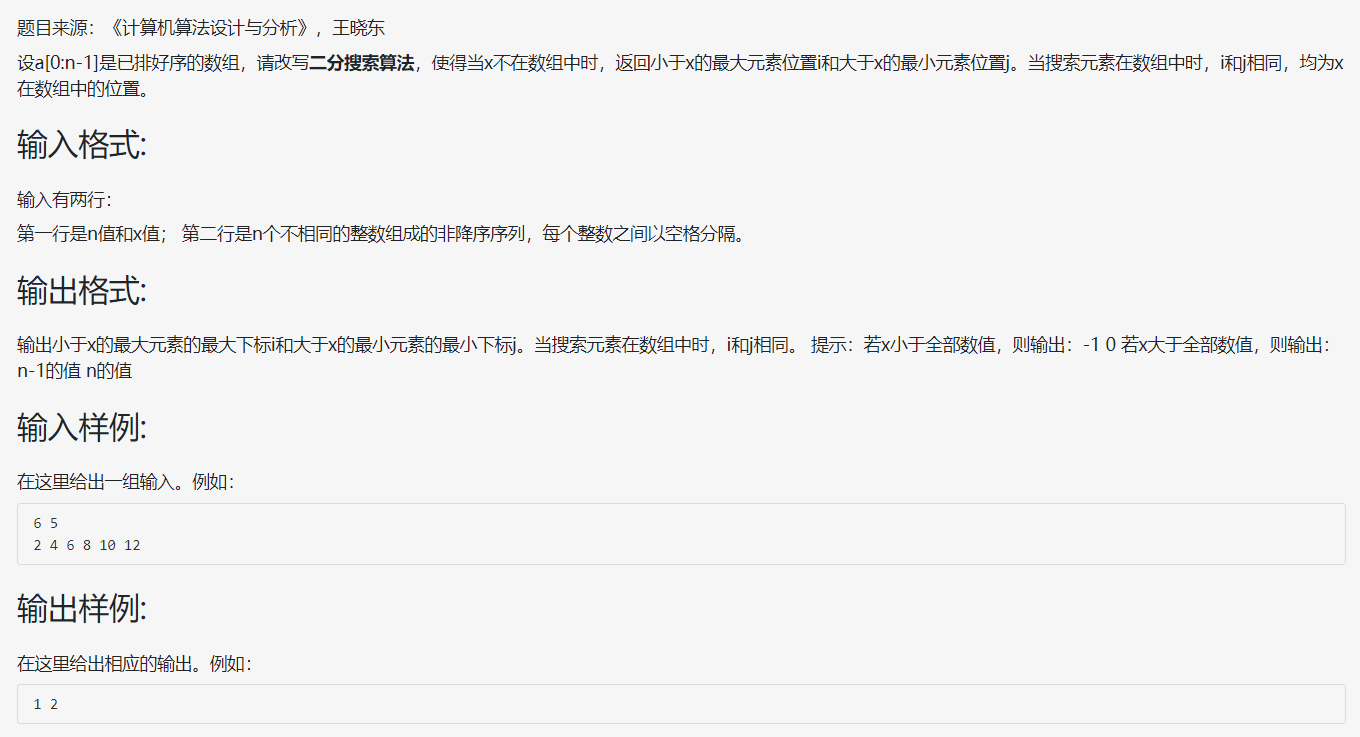代码如下：


#include<iostream>
using namespace std;
int binarySearch(int arr[], int x, int low, int high) {
if (low > high) {
return low;
};
int mid = (low + high) / 2;
if (arr[mid] == x) {
return mid;
};
if (arr[mid] > x) {
return binarySearch(arr, x, low, mid - 1);
}
else {
return binarySearch(arr, x, mid + 1, high);
}
}

int main() {
int len;
int arr;
int x;
cin >> len;
cin >> x;
for (int i = 0; i < len; i++) {
cin >> arr[i];
};
int index = binarySearch(arr, x, 0, len - 1);
if (arr[index] == x) {
cout << index << " " << index << endl;
}
else {
cout << index - 1 << " " << index << endl;
}
system("pause");
return 0;
}


解析：
这道题我们需要输出x不在数组中时，返回小于x的最大元素位置i和大于x的最小元素位置j。只要对经典二分查找算法binarySearch函数进行改写，当元素不存在时，返回low即可。（图用left和right更明显）。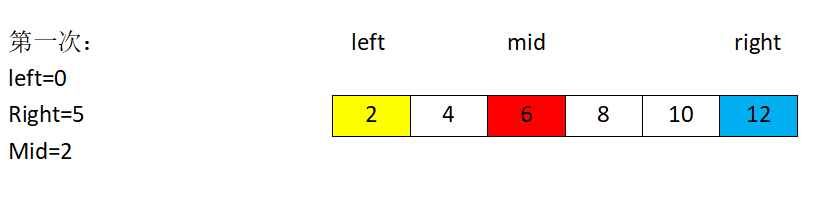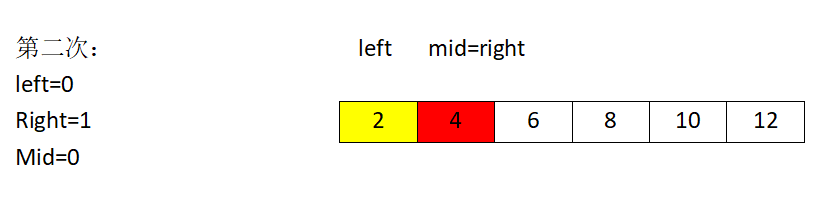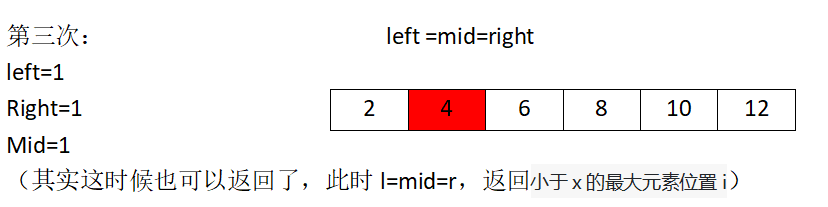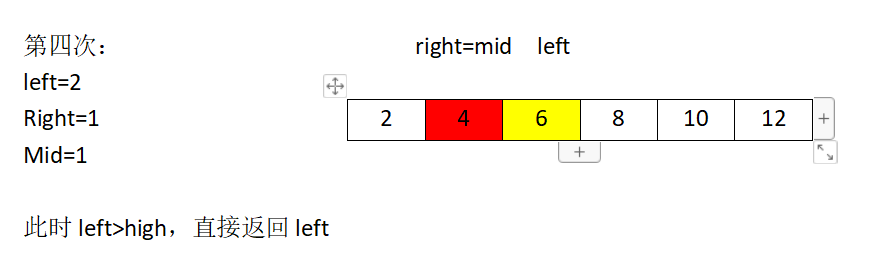因为当low>high时即退出递归返回，所以此时low一定是刚好大于x的最小元素位置的下标（即j）。由题目可以看出，i和j的坐标一定是连续的。所以只要输出low和low-1即可。当元素存在时，返回mid下标并输出。

展开全文C++ 分治法
• C++ 改写二分搜索算法 题目来源：《计算机算法设计与分析》，王晓东 设a[0:n-1]是已排好序的数组，请改写二分搜索算法，使得当x不在数组中时，返回小于x的最大元素位置i和大于x的最小元素位置j。当搜索元素在数组中...C++
• 【计算机算法改写二分搜索算法 题目来源：《计算机算法设计与分析》，王晓东 设a[0:n-1]是已排好序的数组，请改写二分搜索算法，使得当x不在数组中时，返回小于x的最大元素位置i和大于x的最小元素位置j。当搜索...
• 改写二分搜索算法 7-2改写二分搜索算法(20分) 题目来源：《计算机算法设计与分析》，王晓东 设a[0:n-1]是已排好序的数组，请改写二分搜索算法，使得当x不在数组中时，返回小于x的最大元素位...
• 改写二分搜索算法，使得当搜索元素x不在数组中时，返回小于x的最大元素位置i和大于x的最小元素位置j。当搜索元素在数组中时，i和j相同，均为x在数组中的位置。 void BinarySearch(int a[],int x,int left,int ...
• 设a[0:n-1]是已排好序的数组，请改写二分搜索算法，使得当x不在数组中时，返回小于x的最大元素位置i和大于x的最小元素位置j。当搜索元素在数组中时，i和j相同，均为x在数组中的位置。问题来源：题目来源：《计算机...设计 c++
• import java.util.Scanner; public class di { public static void main(String[] args) { // TODO Auto-generated method stub Scanner s=new Scanner(System.in); int n=s.nextInt();... int x=s.nextInt();...数据结构 java
• 改写二分搜索算法，使得当搜索元素x不在数组中时，返回小于x的最大元素位置i和大于x的元素位置j。当搜索元素在数组中时，i和j相同，均为x在数组中的位置。 //二分查找 #include <bits/stdc++.h> using ...c++
• 设a[0:n-1]是已排好序的数组，请改写二分搜索算法，使得当x不在数组中时，返回小于x的最大元素位置i和大于x的最小元素位置j。当搜索元素在数组中时，i和j相同，均为x在数组中的位置。 输入格式: 输入有两行： 第一行...
• 改写二分搜索算法，使得当搜索元素x不在数组中时，返回小于x的最大元素的位置I和大于x的最大元素位置j public static int binarySearch(int []a,int x,int n) {int left=0; int right=n-1; int i=j=0; while...
• 设a[0:n-1]是已排好序的数组，请改写二分搜索算法，使得当x不在数组中时，返回小于x的最大元素位置i和大于x的最小元素位置j。当搜索元素在数组中时，i和j相同，均为x在数组中的位置。 代码： #include&lt;...
• 一、实践题目：改写二分搜索算法 二、问题描述 设a[0:n-1]是已排好序的数组，请改写二分搜索算法，使得当x不在数组中时，返回小于x的最大元素位置i和大于x的最小元素位置j。当搜索元素在数组中时，i和j相同，均为x在...
• 改写二分搜索算法，使得当搜索元素 x 不在数组中时，返回小于 x 的最大元素的位置 i 和大于 x 的最小元素位置 j。当搜索元素在数组中时，i 和 j 相同，均为 x 在数组中的位置。测试数据（自己写的）：61234567输出...
• 主要是对二分法的改写，如果熟悉二分法就非常简单。 具体代码如下： #include&lt;stdio.h&gt; #include&lt;stdlib.h&gt; //二分法查找 int search(int a[],int length, int x) //a是搜索数组，x...
• 改写二分搜索算法，使得当搜索元素x不在数组中时，返回小于x的最大元素的位置i和大于x的最小元素位置j。当搜索元素在数组中时，i和j相同，均为x在数组中的位置。并对自己的程序进行复杂性分析。 #include <...数据结构
• 算法分析 二分搜索算法 实验报告 包含实验目的 试验分析和程序代码
• 改写二分搜索算法，使得当搜索元素x不在数组a中时，返回小于x的最大元素的位置i和大于x的最小元素的位置j；当搜索元素x在数组a中时，返回x在数组中的位置，此时i和j相同。 ** 代码如下 ** def binary_search(arr_...
• 改写二分搜索算法，当搜索元素x不在数组中时，返回小于x的最大元素的位置i和大于x的最小位置j。当搜索元素在数组中时，i和j相同，均为x在数组中的位置。代码：#include&lt;stdio.h&gt;void main(){int a......